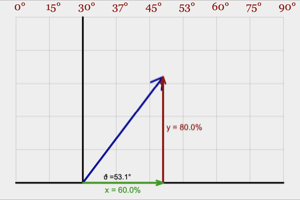Programs for 2nd Law (Net Force)
Below are all the programs that might be used with this topic. Click on "See Resources" to see if there are any student directions, lab sheets or other materials that have been created for this program.
Pulleys

Lab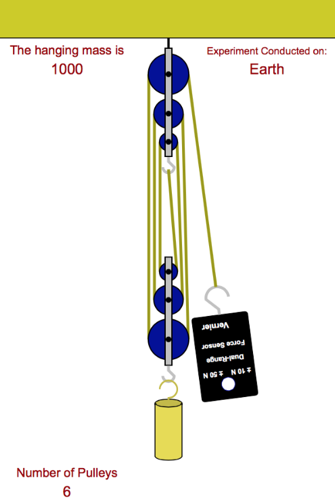Force Net Difficult

Homework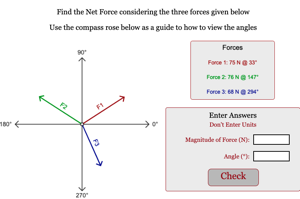Static Equilibrium Level 1

HomeworkStatic Equilibrium Level 2

HomeworkStatic Equilibrium Level 3

HomeworkResultant Vector

HomeworkOne Step Vector Treasure Hunt Game

GameVector Treasure Hunt Game

GameNon-Linear Net Force Game

Game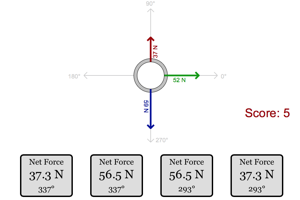Linear Net Force Game

Game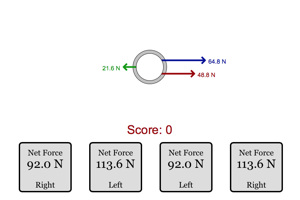Triangle Math Challenge

Game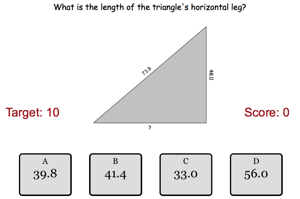Trig Stuff

Tool